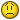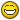# [Help] What wrong in Fix defun ?## Recommended PostsI've a problem with this function (in my case it isn't have answer)

```(defun test (a)
(setq b (atof a))
(setq c (fix B))
(setq d (rtos C 2 0 ))
(setq e (- b (fix B)))
(setq e1 (* e 100))
(setq e2 (fix e1))
(setq e3(rtos e2 2 0))
(setq g (- e1 (fix e1)))
(setq g1 (* g 100))
(setq g2 (fix g1))
(setq g3(rtos g2 2 0))
(princ (strcat d " d "  e3 " m " g3 " s "))(princ)
)```

Everything seem to be Ok, but, when i test with some string with 00 in last, it return wrong.

Ex

Command: (test "45.2300") ;want it return 45 23 00

45 d 22 m 99 s ;but it return this

;So I check value in progress

Command: !b

45.23

Command: !c

45

Command: !e

0.23

Command: !e1

23.0

Command: !e2

22

Command: !e3

"22"

Command: !g

1.0

Command: !g1

100.0

Command: !g2

99

Command: !g3

"99"

Why e1 return 22 and g2 return 99 ?

Many thanks all ^^

##### Share on other sitesBecause of the rounding of Doubles, perhaps look into the angtof/angtos functions.

##### Share on other sitesI just guess it cause of Rounding, but don't know what happend in progress calculate ^^ So i worry about making the same problem in other defun, not only in Degree convert...

##### Share on other sitesMore fun in Fix ^^

Command: (- 3.2 (fix 3.2) 0.2)

1.66533e-016

##### Share on other sitesYep, try to stear clear of using the fix function - there's some issues when mixing integers & floating point numbers. I'm not exactly sure why you want to do this - it's as if you simply type in the dms, but with the wrong notation. If you perform the next: (angtos (angtof "45.2300") 1) ... you get a value of 45d13'48" since your entry is in decimal 45 + 23/100 (instead of 45 + 23/60).

Anyway, try this one:

```(defun testang (str / d)
(if (setq d (vl-string-search "." str))
(setq str (strcat (substr str 1 d) "d" (substr str (+ d 2) 2) "'" (substr str (+ d 4)) "\""))
)
(angtos (angtof str) 1)
)```

You could actually remove the last line. I.e. you simply manipulate the string. In which case you could simply have done this to get exactly "45 23 00":

```(defun testang (str / d)
(if (setq d (vl-string-search "." str))
(setq str (strcat (substr str 1 d) " " (substr str (+ d 2) 2) " " (substr str (+ d 4))))
(strcat str " 00 00")
)
)```

Or one which works a bit better if they "forget" to enter the minutes / seconds:

```(defun testang (str / d emtystring->00)
(defun emtystring->00 (str)
(if (or (not str) (eq str ""))
"00"
str
)
)
(if (setq d (vl-string-search "." str))
(setq str (strcat (substr str 1 d)
" "
(emtystring->00 (substr str (+ d 2) 2))
" "
(emtystring->00 (substr str (+ d 4)))
)
)
(strcat str " 00 00")
)
)```

##### Share on other sitesMany Thanks irnebI can solve it by string functions, but I still problem with "what wrong with FIX" in #4 post. I don't know what exactly problem in hereand i'm so vagueANW, should i forgot it to do something else ^^

##### Share on other sitesbut I still problem with "what wrong with FIX" in #4 post.

There's nothing 'wrong' with the fix function. Consider that numbers cannot be expressed to an inifinite number of decimal places as they are in mathematics (since this would obviously require an infinite amount of memory), hence some calculations can lose some degree of accuracy.

Note that your result of 1.66533e-016 is extremely small and is indeed on the limit of accuracy for the IEEE 754 double-precision floating-point format (double) wherein a number can be approximated to about 16 decimal places.

When equating values of doubles (in conditional statements, for example) always use the equal function with a small tolerance to compensate for this rounding effect.

##### Share on other sitesAs a method to show what's actually happening, consider showing each variable using as much precision as possible:

```(rtos b 1 16) ;--> "4.522000000000000E+01"
(rtos e 1 16) ;--> "2.199999999999988E-01"```

Already you can see an error occurring. Though extremely small, this starts accumulating the more you use the value in more calculations. And since you keep multiplying and then fix the result to get the next 2 digits, this gets worse and worse.

In computer terms these errors "shouldn't" occur as long as all operations and values are in powers of 2. Since then it would simply be a shift in position. But seeing as many of your calculations work in powers of 10 a simple x10 is not a "simple" binary calculation. And since there's only a finite amount of bits to store this number, once it gets to the point where all the bits are needed the "so-called" least-significant-bits are dropped.

The fix function doesn't account for this, since a fix of 21.99999999999999988 would still only give 21. It doesn't round off to the nearest integer, it simply cuts off the floating point piece. You could use a round-off function of your own instead of fix (there's no standard one in ALisp though), or you could convert to and from strings all the time - though that has other problems as well, and simply passes the error along while possible causing worse.

##### Share on other sitesThanks all Lee and irneb, it' cleared my mind . Have a good day( i don't know how to say alot of "THank you " because of limiting in my English )

## Join the conversation

You can post now and register later. If you have an account, sign in now to post with your account.
Note: Your post will require moderator approval before it will be visible.×   Pasted as rich text.   Restore formatting

Only 75 emoji are allowed.